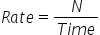Mathematics
Easy

Question

# Nadia scored 12 touchdowns in her first 6 minutes. ___________ touchdowns can be made by her in 20 minutes at the same rate.

## 60        904070Hint:

## The correct answer is: 40

### The given value of touchdowns is 12 and time required for 12 touchdowns is 6 minutes.Let’s denote the number of touchdowns by N.N = 12Time = 6 minutesWe have to find the number of touchdowns in 20 minutes. For that, we will find the ratio of number of touchdowns to time. We will get the rate by taking the ratio. As rate is constant, we will use it to find the required value.Let’s find the rate..Now let’s use the rate to find number of touchdowns in 20 minutesLet’s rearrange the equation for N. We will multiply both the sides by N.Rate × Time = NN = Rate × Time= 2 × 20= 40 touchdownsTherefore, Nadia scores 40 touchdowns in 20 minutes.

The other method is to take the ratios and directly equate them. As the rate is constant, so the ratios will be constant. So we will consider the quantity we have to find as x and then equate the ratios. It will be a shortcut.

### Related Questions to study#### With Turito Foundation.#### Get an Expert Advice From Turito.